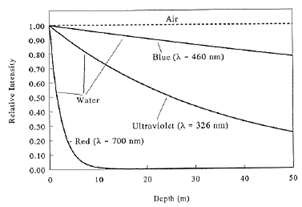# Problem: The graph shows the intensity of transmitted light of different colors (wavelengths) as a function of depth x in meters for absorption in water. Approximately what is the absorption coefficient α for UV (ultraviolet) LIGHT, given the exponential transmission I(x) = I0e−αx ? A. 2 m-1 B. 10-6 m-1 C. 0.003 m-1 D. 50 m-1 E. 0.025 m-1

###### FREE Expert Solution
88% (346 ratings)
###### Problem Details

The graph shows the intensity of transmitted light of different colors (wavelengths) as a function of depth x in meters for absorption in water. Approximately what is the absorption coefficient α for UV (ultraviolet) LIGHT, given the exponential transmission I(x) = I0e−αx ?

A. 2 m-1

B. 10-6 m-1

C. 0.003 m-1

D. 50 m-1

E. 0.025 m-1